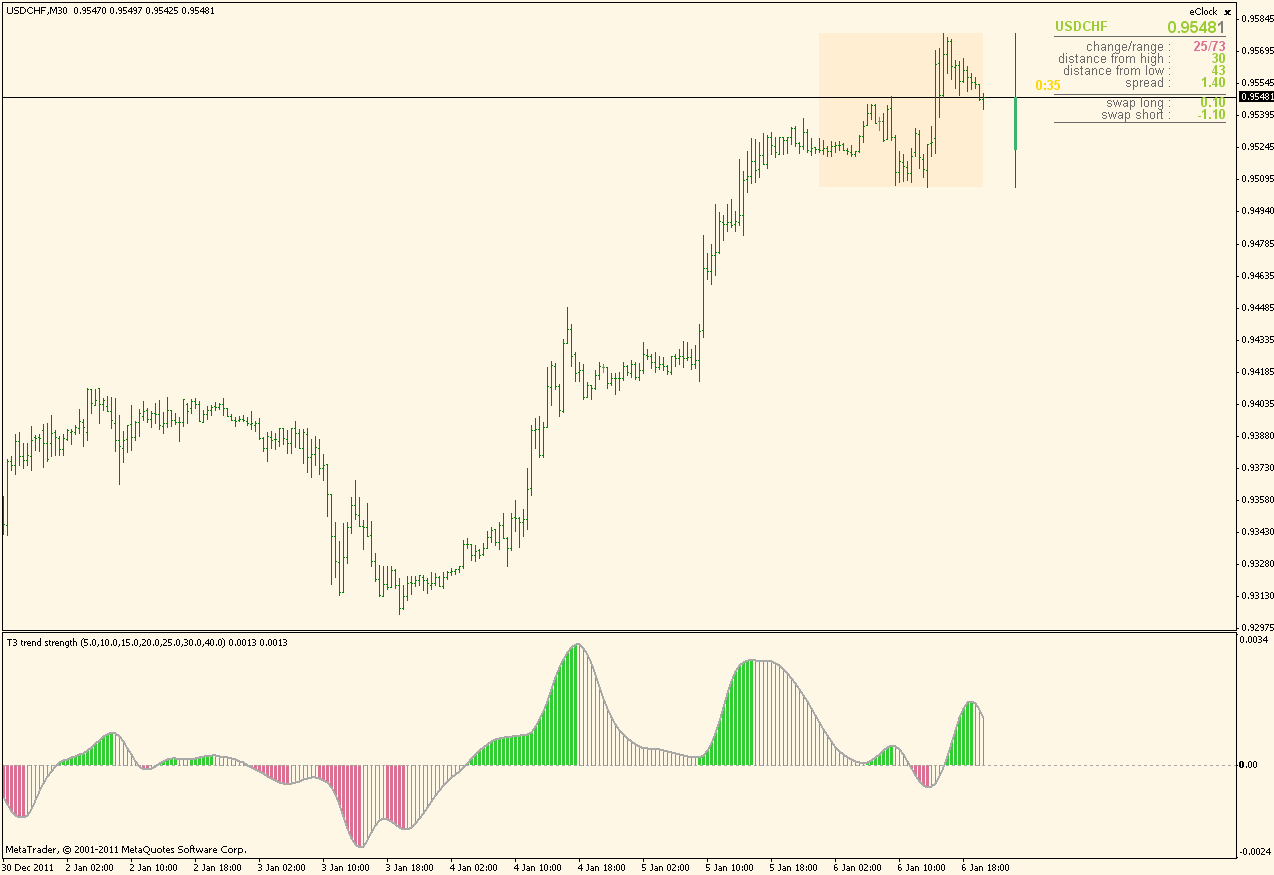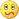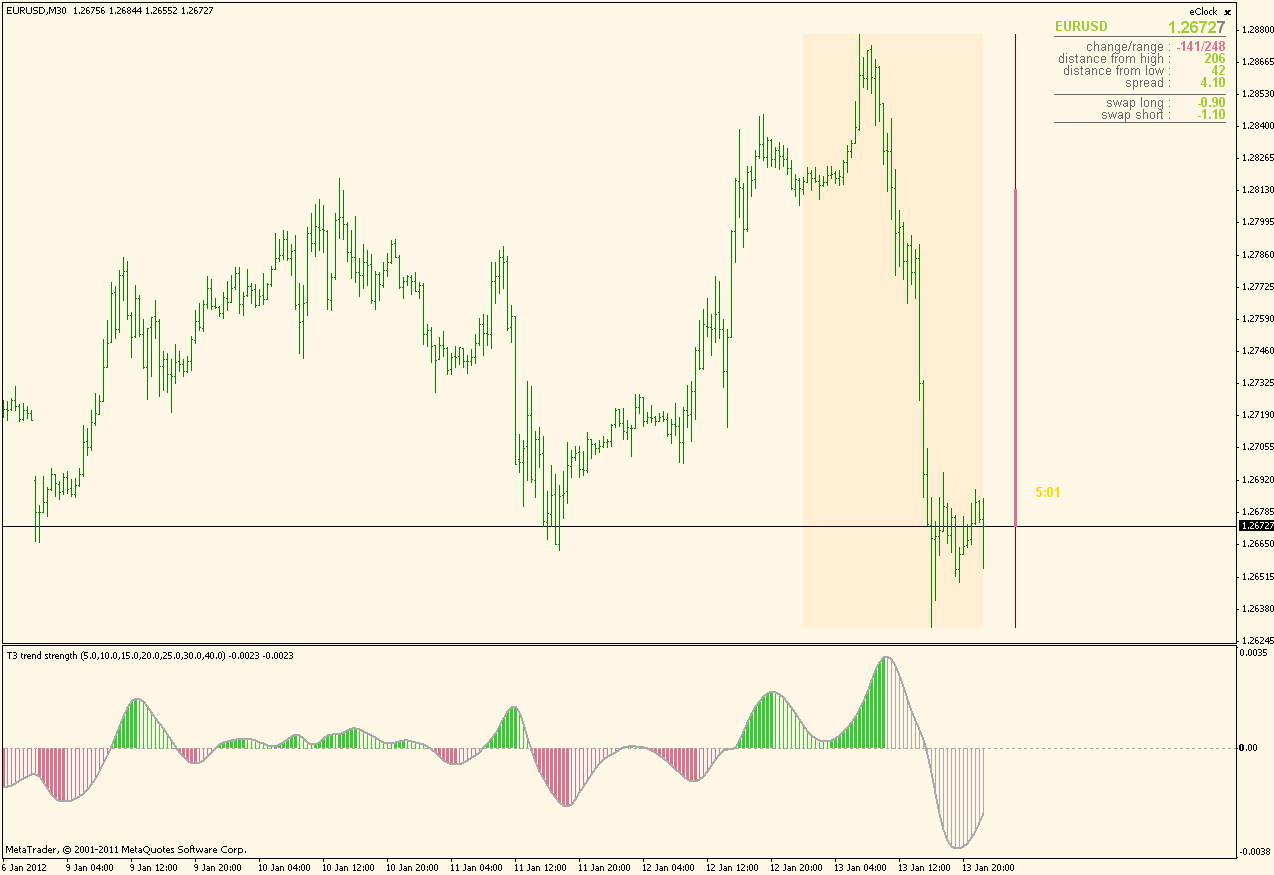# T3 - page 1623

t3 angel !

hello can i ask if there is an indicator for t3 the measures angles in histogram or just line on chart but change color when the needed angel is current ? thx216611

T3 trend strength ...

This is a variation on the trend strength ema ...

It belongs to a family of "composite" indicators (combining more than one basic indicator into one value, a kind of a macd in this case)

Instead of using EMA for calculation, this one uses T3. T3 can be used in 2 variations : Tim Tillson original (T3Original parameter set to true) or Fulks/Matulich version (T3Original parameter set to false - default setting since the Fulks/Matulich version compensates T3 lag significantly compared to original T3). Periods in this one can be set individually (if you want to omit some period, set it to 0) and can be fractional (there is no reason whatsoever why T3 period should be strictly an integer number)Files:
ts_t2.gif  22 kb104

T3 multicross mtf arrows with shift- please

fxbs:
double T3Fast & Hot (mladen)

Could we please have T3 crossover arrows with a shift for each T3- I tried but just made a mess.

If someone want's to get industrious-

I would like it to have-

6 shiftable T3's each with it's own TF and 3 sizes of arrows 1 for each pair

and a

"price crossover mode" = (1)closed, (2)open, (3)off; that will show a yellow round arrow when price crosses any/all selectable T3 ma's.104

mladen:
This is a variation on the trend strength ema ...

It belongs to a family of "composite" indicators (combining more than one basic indicator into one value, a kind of a macd in this case)

Instead of using EMA for calculation, this one uses T3. T3 can be used in 2 variations : Tim Tillson original (T3Original parameter set to true) or Fulks/Matulich version (T3Original parameter set to false - default setting since the Fulks/Matulich version compensates T3 lag significantly compared to original T3). Periods in this one can be set individually (if you want to omit some period, set it to 0) and can be fractional (there is no reason whatsoever why T3 period should be strictly an integer number)The T3 trend strength is beautiful- a few ideas-maybe they make sense?

Could it have shifts for each ma please?

Does it use price crossing uncrossed ma's as a factor or is it just crossed ma's?

Could we have an "amplfier" factor of some kind to boost the trend strength signal when price crosses a T3 with a closed/unclosed period option?216611

...

To explain a bit more how the "trend strength" of T3 trend strength indicator is calculated.

It is calculating (adding up) 7 differences between a "basic" T3 (the one defined with T3MainPeriod) and the other seven T3 values, and then averages that sum. So, overall, it is an average difference of 7 pairs of T3 (or, as I said a kind of a "composite T3 macd"). It is the simplified description

Difference of each and every T3 and main T3 can be even different in sign, it can have rather complex "sub-differences" and that is resulting in a rater fast reaction in a volatile times.

_____________________________________

In Tim Tillsons original T3 calculation there was what he called a "volume factor" even though it has nothing with volume. The best description I have found is "where "v" is volume factor, which determines how hot the moving averages response to linear trends will be. The author advises to use v=0.7." Instead of "volume" it is common to use "hot". With the explanation from above I think it is much clearer what does the "hot" serve for. In general, the bigger the hot field is the faster the T3 is (and that way you can control the "speed" of it)

_____________________________________

As of shift : it could be done but then, if they were unequal, there could happen cases when a difference of something and nothing should be calculated (some shifts would cause lack of calculated data). Hence, it is easy t shift the whole thing by same shift, but shifting each and every T3 by some different value would cause the indicator stop working in some cases

Hope this clarifies a bit what exactly T3 trend strength does

angrysky:
The T3 trend strength is beautiful- a few ideas-maybe they make sense?

Could it have shifts for each ma please?

Does it use price crossing uncrossed ma's as a factor or is it just crossed ma's?

Could we have an "amplfier" factor of some kind to boost the trend strength signal when price crosses a T3 with a closed/unclosed period option?104

mladen:
To explain a bit more how the "trend strength" of T3 trend strength indicator is calculated.

It is calculating (adding up) 7 differences between a "basic" T3 (the one defined with T3MainPeriod) and the other seven T3 values, and then averages that sum. So, overall, it is an average difference of 7 pairs of T3 (or, as I said a kind of a "composite T3 macd"). It is the simplified description

Difference of each and every T3 and main T3 can be even different in sign, it can have rather complex "sub-differences" and that is resulting in a rater fast reaction in a volatile times.

_____________________________________

In Tim Tillsons original T3 calculation there was what he called a "volume factor" even though it has nothing with volume. The best description I have found is "where "v" is volume factor, which determines how hot the moving averages response to linear trends will be. The author advises to use v=0.7." Instead of "volume" it is common to use "hot". With the explanation from above I think it is much clearer what does the "hot" serve for. In general, the bigger the hot field is the faster the T3 is (and that way you can control the "speed" of it)

_____________________________________

As of shift : it could be done but then, if they were unequal, there could happen cases when a difference of something and nothing should be calculated (some shifts would cause lack of calculated data). Hence, it is easy t shift the whole thing by same shift, but shifting each and every T3 by some different value would cause the indicator stop working in some cases

Hope this clarifies a bit what exactly T3 trend strength does

Could we optionally shift every other T3?

Maybe a shift limit or way to smooth 0 calculation periods with a higher period?

Could we please have a dot or up/down arrow on the indie when the main T3 ma is crossed (open/closed option might be nice)?

I am really after the T3 arrows with a shift option.

Thanks,

Ty9557

Hi Ty,

This is not the T3 Trend Strength, but just 2 regular T3 crossing, arrows indicator with shift option, so not sure if you can use it.

Files:
t3ma_shift.gif  20 kb104

mrtools:
Hi Ty, This is not the T3 Trend Strength, but just 2 regular T3 crossing, arrows indicator with shift option, so not sure if you can use it.

Thank you very much.

For some reason I can't use exactly the same period (which I would)- can I cut some code and get it to let me?9557

angrysky:
Thank you very much. For some reason I can't use exactly the same period (which I would)- can I cut some code and get it to let me?

Don't know216611

...

Here is a T3 strength that with some changes

It allows up to 15 T3 averages to be calculated now (parameters are reorganized, but I think that it is easier this way to use it). Also, each and every T3 can have it's own shift. Because of that appearance of the indicator can change in cases when any of the calculated T3 averages has a negative shift. A problem with negative shift is that it addresses future prices and that at the end of data (meaning when it is near current prices) it lacks prices (they simply do not exist yet). So, if there is any negative shift in any T3 calculation it means that the "shift" period is going to be changed (recalculated, repainted whichever expression one prefers) when the price is in. For that reason indicator draws that period in gray color now indicating that that part of calculation is a subject of change

Here is an example in which 0 T3 shift is set to -25 (I used a big negative shift on one f the t3 shifts in order to make it clearly visible hat is happening in those cases): the last 25 bars are drawn in gray since as the new prices are formed, that part of indicator will be changedt3_trend_strength_2.mq4

PS: for positive shift there is no such an issue (since they always are using past prices)

Files:
t3_ts_2.gif  27 kb